# Communication Patterns in Cloud Haskell (Part 4)

## K-Means

In Part 3 of this series we showed how to write a simple distributed implementation of Map-Reduce using Cloud Haskell. In this final part of the series we will explain the K-Means algorithm and show how it can be implemented in terms of Map-Reduce.

K-Means is an algorithm to partition a set of points into n clusters. The algorithm iterates the following two steps for a fixed number of times (or until convergence):

• Given a set of points and n cluster centres, associate each point with the cluster centre it is nearest to.
• Compute the centre of each new cluster.

The initial cluster centres can be chosen randomly. Here is one example run of the first 5 iterations of the algorithm on randomly (evenly) distributed two-dimensional points: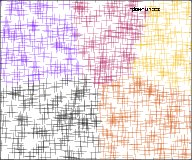Of course, in such an evenly distributed data set the clusters that are "discovered" are more or less arbitrary and heavily influenced by the choice of initial centers. For example, here is another run of the algorithm with different initial centers: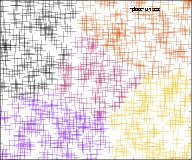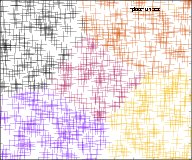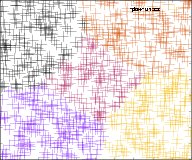## K-Means as a Map-Reduce skeleton

We will use Map-Reduce to implement a single iteration of the K-Means algorithm. Each mapper node will execute step (1) of the algorithm for a subset of the points, and in the reduction step we will compute the new cluster centres.

```type Point    = (Double, Double)
type Cluster  = (Double, Double)

average :: Fractional a => [a] -> a
average xs = sum xs / fromIntegral (length xs)

distanceSq :: Point -> Point -> Double
distanceSq (x1, y1) (x2, y2) = a * a + b * b
where
a = x2 - x1
b = y2 - y1

nearest :: Point -> [Cluster] -> Cluster
nearest p = minimumBy (compare `on` distanceSq p)

center :: [Point] -> Point
center ps = let (xs, ys) = unzip ps in (average xs, average ys)

kmeans :: Array Int Point -> MapReduce (Int, Int) [Cluster] Cluster Point ([Point], Point)
kmeans points = MapReduce {
mrMap    = \(lo, hi) cs -> [ let p = points ! i in (nearest p cs, p)
| i <- [lo .. hi]
]
, mrReduce = \_ ps -> (ps, center ps)
}
```

We start with a `Map (Int, Int) [Cluster]`; the keys in this map correspond to the segmentation of the input set; for instance, a key `(20, 39)` indicates that a mapper node should compute clusters for points `[20 .. 39]`. The values in this map are the current center (that is, every key in the map has the same value).

The mappers then compute a list `[(Cluster, Point)]` which associates points with each cluster. Finally, in the reduction step we create a ```Map Cluster ([Point], Point)``` which tells us for each cluster its set of points and its centre.

## Iterating locally

The Map-Reduce skeleton only computes a single iteration of the algorithm; we need to iterate this a number of steps to implement the full algorithm. Using the `localMapReduce` we defined above we can do this as

```localKMeans :: Array Int Point
-> [Cluster]
-> Int
-> Map Cluster ([Point], Point)
localKMeans points cs iterations = go (iterations - 1)
where
mr :: [Cluster] -> Map Cluster ([Point], Point)
mr = localMapReduce (kmeans points) . trivialSegmentation

go :: Int -> Map Cluster ([Point], Point)
go 0 = mr cs
go n = mr . map snd . Map.elems . go \$ n - 1

trivialSegmentation :: [Cluster] -> Map (Int, Int) [Cluster]
trivialSegmentation cs' = Map.fromList [(bounds points, cs')]
```

For the local implementation we don't care about how we partition the input, so we just create a trivial segmentation and have one mapper process the entire input.

## Generalizing the distributed Map-Reduce implementation

The set of points itself does not vary from one iteration of the algorithm to another, and only needs to be distributed to the mapper nodes once. The master process of our Map-Reduce implementation from Part 3 however looks like

• Initialize the mappers
• Run the Map-Reduce query
• Terminate the mappers

This means that if we use `distrMapReduce` as implemented we will re-distribute the full set of points to the mapper nodes on every iteration of the algorithm. To avoid this, we can generalize the Map-Reduce implementation to be

• Initialize the mappers
• Run as many Map-Reduce queries as necessary
• Terminate the mappers

We will change the type of `distrMapReduce` to

```distrMapReduce :: Closure (MapReduce (Point, Point) [Cluster] Cluster
Point ([Point], Point))
-> [NodeId]
-> ((Map (Point, Point) [Cluster] ->
Process (Map Cluster ([Point], Point))) -> Process a)
-> Process a
```

In `distrMapReduce mrClosure mappers p` the process `p` is provided with a means to run map-reduce queries; compare the type of `distrMapReduce` to the types of functions such as

```withFile :: FilePath -> IOMode -> (Handle -> IO r) -> IO r
```

Exercise 8: Implement `distrMapReduce` with the type above. This does not require any new Cloud Haskell concepts, but does require a bit of engineering. (You can find the implementation also in the `distributed-process-demos` package).

Note that even with this generalization we will pass the entire set of points to all the nodes, even though each node will only operate on a subset of them; we leave optimizing this as an exercise to the reader (it will require a further generalization of the Map-Reduce driver).

## Polymorphism

In the section above we changed the type of `distrMapReduce` to match the type of the K-Means MapReduce skeleton instead of the word-counting MapReduce skeleton; we can change that type without changing the implementation at all. What we really want, of course, is a polymorphic implementation of Map-Reduce:

```distrMapReduce :: (Serializable k1, Serializable v1, Serializable k2,
Serializable v2, Serializable v3, Ord k2)
=> Closure (MapReduce k1 v1 k2 v2 v3)
-> [NodeId]
-> ((Map k1 v1 -> Process (Map k2 v3)) -> Process a)
-> Process a
```

However, when we try to generalize our code above we run into a problem. Consider the code that we ship to the mapper nodes. What does this code need to do? First, it needs to wait for a message of a specific type. In order to do the type matching it needs some information about the type `(k1, v1)`. Once it receives such a message, it needs to send the list of type `[(k2,v2)]` created by the Map function back to the master. In order to do that, it needs to know how to serialize values of type `[(k2,v2)]`.

Where does `distrMapReduce` get this information? Well, it is provided by the `Serializable` type class constraints. Unfortunately, however, Haskell does not give an explicit handle on these arguments, much less provide us with a way to serialize these arguments so that we can ship them to the mapper nodes. We can, however, reify a type class constraint as an explicit dictionary:

```data SerializableDict a where
SerializableDict :: Serializable a => SerializableDict a
```

We cannot serialize objects of type `SerializableDict` directly, but we can serialise static `SerializableDict`s! Hence, the type of `distrMapReduce` becomes:

```distrMapReduce :: forall k1 k2 v1 v2 v3 a.
(Serializable k1, Serializable v1, Serializable k2,
Serializable v2, Serializable v3, Ord k2)
=> Static (SerializableDict (k1, v1))
-> Static (SerializableDict [(k2, v2)])
-> Closure (MapReduce k1 v1 k2 v2 v3)
-> [NodeId]
-> ((Map k1 v1 -> Process (Map k2 v3)) -> Process a)
-> Process a
```

This type may look a bit intimidating, but really all that has changed is that we require static type information so that we can ship this type information to the mappers. We omit the implementation; you can find it in the `distributed-process-demos` package; the general principles are explained in the documentation of the distributed-static package.

Using this polymorphic version of `distrMapReduce` is no more difficult than using the monomorphic version; for example, we can implement "distributed word counting" as

```dictIn :: SerializableDict (FilePath, Document)
dictIn = SerializableDict

dictOut :: SerializableDict [(Word, Frequency)]
dictOut = SerializableDict

countWords_ :: () -> MapReduce FilePath Document Word Frequency Frequency
countWords_ () = countWords

remotable ['dictIn, 'dictOut, 'countWords_]

distrCountWords :: [NodeId] -> Map FilePath Document -> Process (Map Word Frequency)
distrCountWords mappers input =
distrMapReduce \$(mkStatic 'dictIn)
\$(mkStatic 'dictOut)
(\$(mkClosure 'countWords_) ())
mappers
(\iteration -> iteration input)
```

Creating the necessary `SerializableDict`s is easy (there is only one constructor for `SerializableDict`, after all, and it doesn't take any arguments!). Note that the word counter only calls the `iteration` function once; this will not be true for the final algorithm we consider: distributed k-means.

## Distributed K-Means

The distributed K-means is not much more complicated. Everything up to and including `go` pretty much follows the local implementation; the remainder (`segments`, `dividePoints`, `pointsPerMapper` and `numPoints`) just compute which segment of the input each mapper node is going to do.

```dictIn :: SerializableDict ((Int, Int), [Cluster])
dictIn = SerializableDict

dictOut :: SerializableDict [(Cluster, Point)]
dictOut = SerializableDict

remotable ['kmeans, 'dictIn, 'dictOut]

distrKMeans :: Array Int Point
-> [Cluster]
-> [NodeId]
-> Int
-> Process (Map Cluster ([Point], Point))
distrKMeans points cs mappers iterations =
distrMapReduce \$(mkStatic 'dictIn)
\$(mkStatic 'dictOut)
(\$(mkClosure 'kmeans) points)
mappers
(go (iterations - 1))
where
go :: Int
-> (Map (Int, Int) [Cluster] -> Process (Map Cluster ([Point], Point)))
-> Process (Map Cluster ([Point], Point))
go 0 iteration = do
iteration (Map.fromList \$ map (, cs) segments)
go n iteration = do
clusters <- go (n - 1) iteration
let centers = map snd \$ Map.elems clusters
iteration (Map.fromList \$ map (, centers) segments)

segments :: [(Int, Int)]
segments = let (lo, _) = bounds points in dividePoints numPoints lo

dividePoints :: Int -> Int -> [(Int, Int)]
dividePoints pointsLeft offset
| pointsLeft <= pointsPerMapper = [(offset, offset + pointsLeft - 1)]
| otherwise = let offset' = offset + pointsPerMapper in
(offset, offset' - 1)
: dividePoints (pointsLeft - pointsPerMapper) offset'

pointsPerMapper :: Int
pointsPerMapper =
ceiling (toRational numPoints / toRational (length mappers))

numPoints :: Int
numPoints = let (lo, hi) = bounds points in hi - lo + 1
```

Exercise 9: In this example we create precisely enough segments that every mapper nodes gets a single segment. We could have created more or fewer segments. Why is creating one segment per mapper node the optimal choice for this algorithm? In which case might creating more segments be more efficient?

Exercise 10: The data sent back from the mapper nodes to the master node contains a lot of redundancy. How might you improve that?

## Profiling

To conclude we briefly look at the heap profiles of the master and the slave nodes of our distributed k-means example.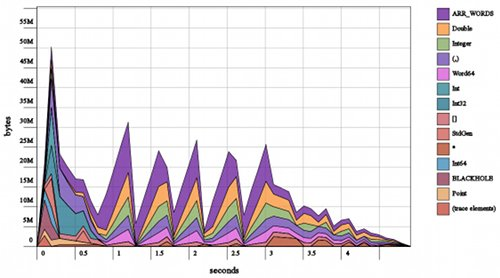Heap profile (by type) of the Map-Reduce master running K-Means Single slave, clustering 50,000 points, 5 iterations

In this example the master process first creates a random set of 50,000 points, before running the K-means algorithm. This is the first peak. Then the other 5 peaks are the master node collecting data from the mapper nodes before reducing them locally.

Exercise 11. In the Work-Stealing example from Part 1 of this series the master reduces as it receives messages (`sumIntegers`). Might you do something similar in the Map-Reduce master?Heap profile (by type) of the Map-Reduce slave running K-Means Single slave, clustering 50,000 points, 5 iterations

In the slave node too, we clearly see the 5 iterations of the algorithm. At the start of each iteration the mapper node creates a list that associates points to clusters. Once the list is created, the list is serialized as a bytestring (purple section in the profile) and sent to the master node before the cycle starts again.

Exercise 12: (More technical) Why is the entire list created before it is serialized to a bytestring? (Hint: look up the `Binary` instance for lists.) How might you change this so that the list is created lazily as it is serialized?